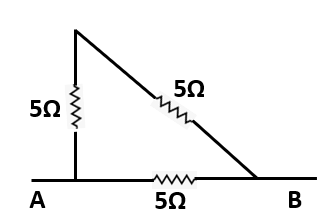Physics
Easy

Question

# Three resistors each of 5 Ω are connected in the form of a triangle. What is the resistance between the vertices?## 3/10 Ω10/3 Ω15/50 Ω2/5 ΩHint:

## The correct answer is: 10/3 Ω

### If three resistors each of 5 Ω are connected in the form of a triangle, the resistance between the vertices is 10/3Ω. The resistances of the vertices in a circuit with the resistors connected in a triangular manner can be calculated as the parallel resistors considering any two corners. If the R1 and R2 are 5 Ω each, the total resistance is 1/5+ 1/10 R= 1/5+1/10= 10/3 Ω#### With Turito Foundation.#### Get an Expert Advice From Turito.